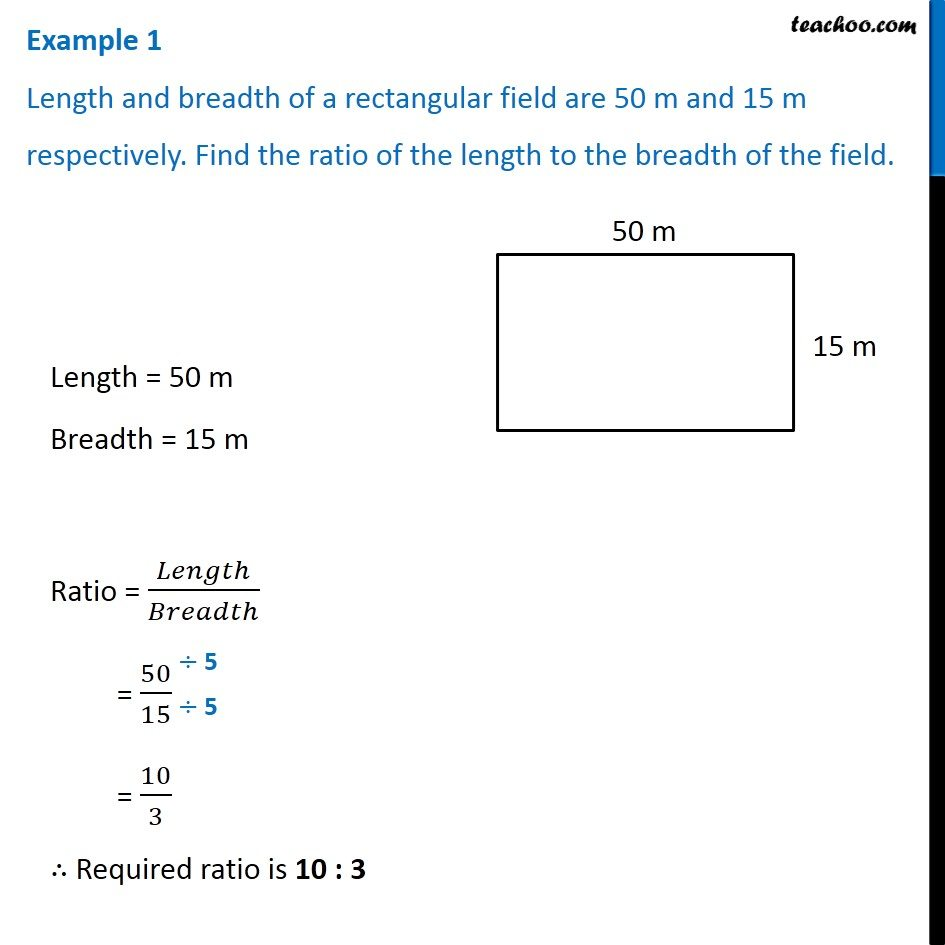Ratios

Chapter 12 Class 6 Ratio and Proportion
Concept wiseLearn in your speed, with individual attention - Teachoo Maths 1-on-1 Class

### Transcript

Example 1 Length and breadth of a rectangular field are 50 m and 15 m respectively. Find the ratio of the length to the breadth of the field. Length = 50 m Breadth = 15 m Ratio = 𝐿𝑒𝑛𝑔𝑡ℎ/𝐵𝑟𝑒𝑎𝑑𝑡ℎ = 50/15 = 10/3 ∴ Required ratio is 10 : 3